# Change Nickname

Current Nickname:
Search
v4.0
v4.0

# k-Means

## Overview

k-Means algorithm classifies all nodes in the graph into k clusters, so the distance from each node to the centroid (geometric center) of its own cluster is shorter than it to the centroid of any other cluster. The distance can calculated by 1) Euclid Distance or 2) Cosine Similarity. The idea of the algorithm dates back to 1957, and the term 'k-means' was proposed by J. MacQueen in 1967. k-Means algorithm is used in vector quantization, clustering analysis, feature learning, computer vision, etc., and is often executed as preprocessing steps for other algorithms.

Related material of the algorithm:

## Basic Concept

### Euclid Distance

In graph, Euclid distance between two nodes is calculated in the N dimensional vector space formed by N properties of node. The formula is as below, please refer to chapter Numerical Similarity for more details.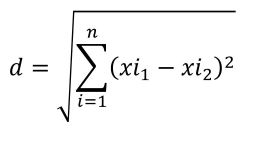### Cosine Similarity

Cosine similarity uses the cosine value of the angle formed by two N-dimensional vectors in vector space to indicate the similarity between them. In graph, the N-dimensional vector space is formed by N node properties, the cosine similarity between two nodes is hence calculated. The formula is as below, please refer to chapter Numerical Similarity for more details.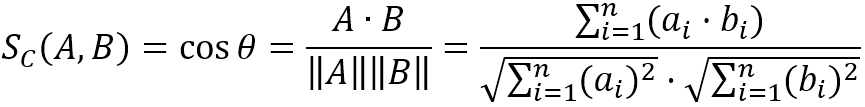### Centroid

The centroid or geometric center of an object in N-dimensional space is the mean position of all the points in all of the coordinate directions. Informally, it is the point at which an infinitesimally thin cutout of the shape could be perfectly balanced on the tip of a pin (assuming uniform density and a uniform gravitational field).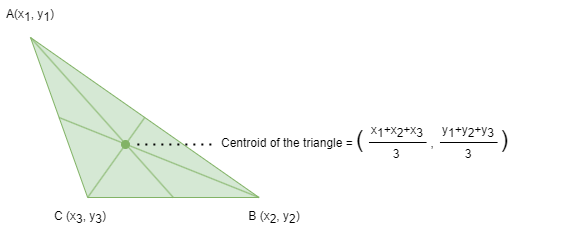A finite number of points always have centroid, which can be obtained by calculating the arithmetic mean ('average') of each coordinate component of these points. The centroid is the only point which has the minimum sum of squares of the distance from one point to this finite number of points in space.

The formula of using Euclid distance to select the centroid of nodes is as below, where `d` is the Euclid distance between the current node `x` and every centroid `m`, select the centroid with the shortest distance as the centroid of the current node `x`: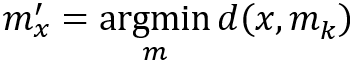The formula of using cosine similarity to select the centroid of nodes is as below, where `s` is the cosine similarity between the current node `x` and every centroid `m`, select the centroid with the maximum similarity as the centroid of the current node `x`: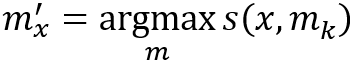Specifying k nodes as the initial centroids of the clusters. From the first iteration, calculate the distance between each node and each centroid, and select the closest centroid for the node; then re-calculate the new centroid for each cluster. The iteration ends when the variation of the clusters meets the preset accuracy requirements, or the number of iterations reaches the limit.

Please note, the selection of the initial centroids would affect the final classification results; if there is more than one centroid have the exact same values of properties, only one of the centroids will take effect while the other equivalent centroid(s) with empty cluster(s).

## Special Case

### Lonely Node, Disconnected Graph

Theoretically, the calculation of Euclid distance or cosine similarity between two nodes does not depend on the existence of edges in the graph. Regardless of whether the two nodes to be calculated are lonely nodes or whether they are in the same connected component, it does not affect the calculation of their Euclid distance or cosine similarity.

### Self-loop Edge

The calculation of k-Means has nothing to do with edges.

### Directed Edge

The calculation of k-Means has nothing to do with edges.

## Results and Statistics

Follow the example above, specifying properties property_1, property_2 and property_3 to form the vector space, measuring by Euclid distance, selecting node 2, 4, 5 as the initial centroids and setting the maximum number of iterations to 3: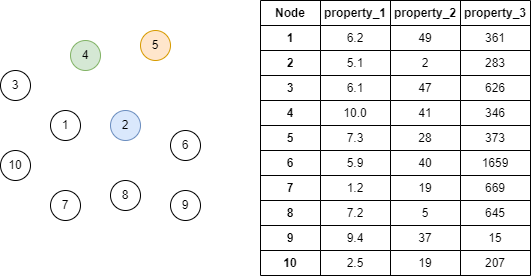Algorithm results: Nodes in the graph are classified in 3 clusters, return `community` and `ids`

community ids
0 9,10,
1 1,2,4,5
2 3,6,7,8,

Algorithm statistics: N/A

## Command and Configuration

• Command: `algo(k_means)`
• Configurations for the parameter `params()`:
Name
Type
Default
Specification
Description
start_ids []`_uuid` / / Specifying k nodes as the initial centroids by UUID, the classification result for centroids with duplicated property values is empty; k initial centroids selected by the system if not set
k int 1 [1, Number of nodes]; mandatory Classify into k clusters
distance_type int 1 1 or 2 Regulate how the distance is calculated, 1 or if not set means by Euclid distance, 2 means cosine similarity
node_schema_property []`@<schema>?.<property>` / Numeric edge property, LTE needed; mandatory At least two node properties that participate in the calculation of distance, schema can be either carried or not; node without any specified property does not participate in the calculation
loop_num int / >=0; mandatory The maximum number of iterations
limit int -1 >=-1 Number of results to return; return all results if sets to -1 or not set

Example: Classify nodes into 3 clusters with k-Means algorithm based on Euclid distance, use property_1, property_2 and property_3 to calculate and specify nodes UUID = 2,4,5 as initial centroids, iterate 4 rounds the maximum

``````algo(k_means).params({
start_ids: [2,4,5],
k: 3,
distance_type: 1,
node_schema_property:["property_1", "property_2", "property_3"],
loop_num: 4
}) as k3 return k3
``````

## Algorithm Execution

#### 1. File Writeback

Configuration Data in Each Row
filename `community`:`_id`,`_id`,...

Example: Classify nodes into 2 clusters with k-Means algorithm based on cosine similarity, use property_1 and property_2 to calculate and specify nodes UUID = 2,5 as initial centroids, iterate 3 rounds the maximum, write the algorithm results back to file named communities

``````algo(k_means).params({
start_ids: [2,5],
k: 3,
distance_type: 2,
node_schema_property: ["property_1", "property_2"],
loop_num: 3
}).write({
file:{
filename: "communities"
}
})
``````

#### 2. Property Writeback

Not supported by this algorithm.

#### 3. Statistics Writeback

This algorithm has no statistics.

### Direct Return

Alias Ordinal Type
Description
Column Name
0 []perCommunity UUID of nodes contained in each cluster `community`, `ids`

Example: Classify nodes into 3 clusters with k-Means algorithm based on Euclid distance, use property_1, property_2 and property_3 to calculate and specify nodes UUID = 2,4,5 as initial centroids, iterate 4 rounds the maximum, define algorithm results as alias named k3, return the results

``````algo(k_means).params({
start_ids: [2,4,5],
k: 3,
distance_type: 1,
node_schema_property:["property_1", "property_2", "property_3"],
loop_num: 4
}) as k3 return k3
``````

### Streaming Return

Alias Ordinal Type
Description
Column Name
0 []perCommunity UUID of nodes contained in each cluster `community`, `ids`

Example: Classify nodes into 3 clusters with k-Means algorithm based on Euclid distance, use property_1, property_2 and property_3 to calculate and specify nodes UUID = 2,4,5 as initial centroids, iterate 4 rounds the maximum, define algorithm results as alias named k3, return the results

``````algo(k_means).params({
start_ids: [2,4,5],
k: 3,
distance_type: 1,
node_schema_property:["property_1", "property_2", "property_3"],
loop_num: 4
}).stream() as k3
return k3
``````

### Real-time Statistics

This algorithm has no statistics.